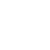SpruceBudworm:
Filter:
Classes (extension) |

# SpruceBudworm : MultiOutUGen : UGen : AbstractFunction : ObjectExtension

Spruce bud worm model equations
Source: SLUGens.sc

//SLUGens released under the GNU GPL as extensions for SuperCollider 3, by Nick Collins, http://composerprogrammer.com/index.html

## Description

One of a number found in A Brief History of Oscillators and Hair Styles of European Men AASU Math/CS Colloquium, April 2002.

Euler ODE solver implementation of a model of foliage and budworm density in Canadian forests ( a logistic model + predation).

x' = k1 x (1-x) - mu*y y' = k2 *y * (1 - (y/alpha*x)) - (rho * (y**2/(beta**2 * x**2 + y**2)))

All inputs can have .kr rate UGens plugged in.

Nonlinear oscillators can blow up, treat with caution.

## Class Methods

### SpruceBudworm.ar(reset: 0, rate: 0.1, k1: 27.9, k2: 1.5, alpha: 0.1, beta: 10.1, mu: 0.3, rho: 10.1, initx: 0.9, inity: 0.1, mul: 1.0, add: 0.0)

#### Arguments:

 reset If > 0.0, restart with new initial conditions sampled from initx, inity rate update rate for a sample step k1 equation constant k2 equation constant alpha equation constant beta equation constant mu equation constant rho equation constant initx reset value for x inity reset value for y